## 手动将浮点 MATLAB 算法转换为定点

• 将算法与测试文件分离。

• 为插桩和代码生成准备算法。

• 管理数据类型并控制位增长。

• 通过创建数据定义表将数据类型定义与算法代码分离。

### 将算法与测试文件分离

```function y = mysum(x) y = 0; for n = 1:length(x) y = y + x(n); end end ```

### 编写测试脚本

1. 编写 MATLAB 脚本 `mysum_test`，它使用双精度数据类型验证您的算法的行为。

```n = 10; rng default x = 2*rand(n,1)-1; % Algorithm y = mysum(x); % Verify results y_expected = sum(double(x)); err = double(y) - y_expected```

`rng default` 将 rand 函数所用的随机数生成器的设置设为其默认值，以便它生成的随机数与重新启动 MATLAB 后生成的随机数相同。

2. 运行测试脚本。

`mysum_test`
```err = 0```

使用 `mysum` 获得的结果与使用 MATLAB `sum` 函数获得的结果相匹配。

### 为插桩和代码生成准备算法

```function y = mysum(x) %#codegen y = 0; for n = 1:length(x) y = y + x(n); end end ```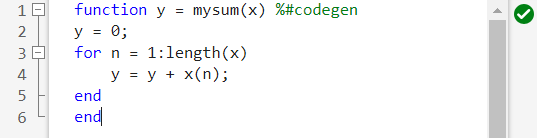### 为原始算法生成 C 代码

1. 将以下行添加到测试脚本的末尾，为 `mysum` 生成 C 代码。

`codegen mysum -args {x} -config:lib -report`

2. 再次运行测试脚本。

MATLAB Coder`mysum` 函数生成 C 代码，并提供代码生成报告的链接。

3. 点击该链接以打开代码生成报告并查看为 `mysum` 生成的 C 代码。

```/* Function Definitions */ double mysum(const double x) { double y; int n; y = 0.0; for (n = 0; n < 10; n++) { y += x[n]; } return y; } ```

由于 C 不允许浮点索引，因此循环计数器 `n` 会自动声明为整数类型。您不需要将 `n` 转换为定点。

输入 `x` 和输出 `y` 声明为双精度类型。

### 管理数据类型和控制位增长

1. 修改您的测试文件，以使 `x` 的数据类型为单精度。

```n = 10; rng default x = single(2*rand(n,1)-1); % Algorithm y = mysum(x); % Verify results y_expected = sum(double(x)); err = double(y) - y_expected codegen mysum -args {x} -config:lib -report```

2. 再次运行测试脚本。

`mysum_test`
```err = -4.4703e-08 ??? This assignment writes a 'single' value into a 'double' type. Code generation does not support changing types through assignment. Check preceding assignments or input type specifications for type mismatches.```

代码生成失败，报告 `y = y + x(n);` 行中的数据类型不匹配。

3. 要查看错误，请打开报告。

在报告的 `y = y + x(n)` 这一行上，以红色突出显示了赋值左侧的 `y`，表明存在错误。存在的问题是：`y` 声明为双精度类型，但被赋了一个单精度类型的值。`y + x(n)` 是双精度和单精度值之和，该和为单精度值。如果将光标置于报告中的变量和表达式上，可以看到有关它们的类型的信息。在此处，您可以看到表达式 `y + x(n)` 是单精度类型。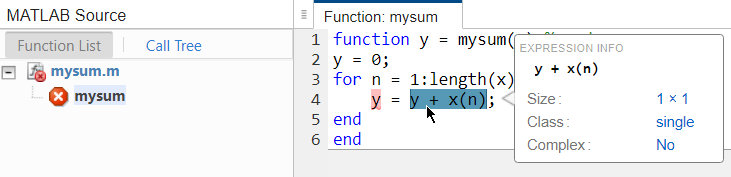4. 要修复类型不匹配，请更新您的算法以对元素之和使用下标赋值。将 `y = y + x(n)` 更改为 `y(:) = y + x(n)`

```function y = mysum(x) %#codegen y = 0; for n = 1:length(x) y(:) = y + x(n); end end ```

使用下标赋值时，您还可以防止位增长，位增长是定点数相加时的默认行为。有关详细信息，请参阅位增长。防止位增长非常重要，因为您要在整个代码中保持定点类型。有关详细信息，请参阅控制位增长

5. 重新生成 C 代码并打开代码生成报告。在 C 代码中，结果现在转换为双精度类型来解决类型不匹配问题。

### 编译插桩后的 MEX

1. 更新测试脚本：

1. 声明 `n` 后，添加 `buildInstrumentedMex mySum —args {zeros(n,1)} -histogram`

2. `x` 更改为双精度类型。用 `x = 2*rand(n,1)-1;` 替换 `x = single(2*rand(n,1)-1);`

3. 调用生成的 MEX 函数，而不是调用原始算法。将 `y = mysum(x)` 更改为 `y=mysum_mex(x)`

4. 调用 MEX 函数后，添加 `showInstrumentationResults mysum_mex -defaultDT numerictype(1,16) -proposeFL``-defaultDT numerictype(1,16) -proposeFL` 标志表示您要为 16 位字长建议小数长度。

这是更新后的测试脚本。

```%% Build instrumented mex n = 10; buildInstrumentedMex mysum -args {zeros(n,1)} -histogram %% Test inputs rng default x = 2*rand(n,1)-1; % Algorithm y = mysum_mex(x); % Verify results showInstrumentationResults mysum_mex ... -defaultDT numerictype(1,16) -proposeFL y_expected = sum(double(x)); err = double(y) - y_expected %% Generate C code codegen mysum -args {x} -config:lib -report ```

2. 再次运行测试脚本。

`showInstrumentationResults` 函数建议数据类型并打开报告以显示结果。

3. 在报告中，点击变量选项卡。`showInstrumentationResults``y` 建议小数长度为 13，对 `x` 建议小数长度为 15。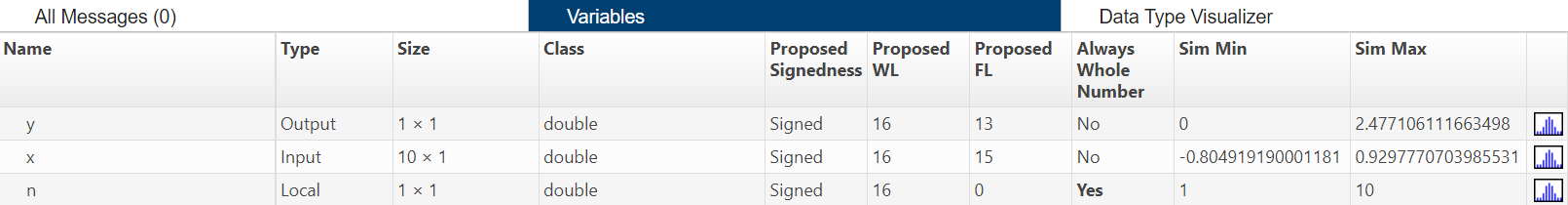• 查看输入 `x` 和输出 `y` 的仿真最小值和最大值。

• 查看对 `x``y` 建议的数据类型。

• 查看代码中所有变量、中间结果和表达式的信息。

要查看此信息，请将光标放在报告中的变量或表达式上。

• 查看 `x``y` 的直方图数据，以帮助您根据当前数据类型识别超出范围或低于精度的任何值。

要查看特定变量的直方图，请点击其直方图图标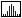### 将数据类型定义与算法代码分离

```function y = mysum(x,T) %#codegen y = cast(0,'like',T.y); for n = 1:length(x) y(:) = y + x(n); end end```

### 创建数据类型定义表

• 使用双精度值来测试数据类型定义表和算法之间的关联。

• 使用单精度值来测试算法以找出数据类型不匹配和其他问题。

• 使用定标双精度值来运行算法以检查是否存在溢出。

```function T = mytypes(dt) switch dt case 'double' T.x = double([]); T.y = double([]); case 'single' T.x = single([]); T.y = single([]); case 'fixed' T.x = fi([],true,16,15); T.y = fi([],true,16,13); case 'scaled' T.x = fi([],true,16,15,... 'DataType','ScaledDouble'); T.y = fi([],true,16,13,... 'DataType','ScaledDouble'); end end```

### 更新测试脚本以使用类型表

1. 对于第一次运行，使用双精度值检查类型表和算法之间的关联。在声明 `n` 之前，添加 `T = mytypes('double');`

2. 更新对 `buildInstrumentedMex` 的调用以使用在数据类型表中指定的 `T.x` 的类型：`buildInstrumentedMex mysum -args {zeros(n,1,'like',T.x),T} -histogram`

3. `x` 转换为使用在表中指定的 `T.x` 的类型：`x = cast(2*rand(n,1)-1,'like',T.x);`

4. 调用传入 `T` 的 MEX 函数：`y = mysum_mex(x,T);`

5. 调用传入 `T``codegen``codegen mysum -args {x,T} -config:lib -report`

以下是更新后的测试脚本。

```%% Build instrumented mex T = mytypes('double'); n = 10; buildInstrumentedMex mysum ... -args {zeros(n,1,'like',T.x),T} -histogram %% Test inputs rng default x = cast(2*rand(n,1)-1,'like',T.x); % Algorithm y = mysum_mex(x,T); % Verify results showInstrumentationResults mysum_mex ... -defaultDT numerictype(1,16) -proposeFL y_expected = sum(double(x)); err = double(y) - y_expected %% Generate C code codegen mysum -args {x,T} -config:lib -report ```

6. 运行测试脚本并点击链接以打开代码生成报告。

生成的 C 代码与为原始算法生成的代码相同。这是因为是使用变量 `T` 指定的类型，且这些类型在代码生成时保持恒定；`T` 在运行时不使用，也不会出现在生成的代码中。

### 生成定点代码

1. 更新测试脚本以使用定点类型。用 `T = mytypes('fixed');` 替换 `T = mytypes('double');`，然后保存该脚本。

2. 运行测试脚本并查看生成的 C 代码。

此 C 代码版本效率不是很高，它包含很多溢出处理。下一步是优化数据类型以避免溢出。

### 优化数据类型

#### 使用定标双精度值来检测溢出

1. 更新测试脚本以使用定标双精度值。用 `T = mytypes('scaled');` 替换 `T = mytypes('fixed');`

2. 再次运行测试脚本。

使用定标双精度值运行测试并显示报告。没有检测到溢出。

到当前为止，您只使用随机输入运行了测试脚本，这意味着测试可能并没有覆盖算法的整个运算范围。

3. 找到输入的完整范围。

`range(T.x)`
```-1.000000000000000 0.999969482421875 DataTypeMode: Fixed-point: binary point scaling Signedness: Signed WordLength: 16 FractionLength: 15```

4. 更新脚本以测试负边界情况。使用原始随机输入和测试整个范围的输入运行 `mysum_mex` 并聚合测试结果。

```%% Build instrumented mex T = mytypes('scaled'); n = 10; buildInstrumentedMex mysum ... -args {zeros(n,1,'like',T.x),T} -histogram %% Test inputs rng default x = cast(2*rand(n,1)-1,'like',T.x); y = mysum_mex(x,T); % Run once with this set of inputs y_expected = sum(double(x)); err = double(y) - y_expected % Run again with this set of inputs. The logs will aggregate. x = -ones(n,1,'like',T.x); y = mysum_mex(x,T); y_expected = sum(double(x)); err = double(y) - y_expected % Verify results showInstrumentationResults mysum_mex ... -defaultDT numerictype(1,16) -proposeFL y_expected = sum(double(x)); err = double(y) - y_expected %% Generate C code codegen mysum -args {x,T} -config:lib -report ```

5. 再次运行测试脚本。

运行测试后，`y` 的值会溢出定点数据类型的范围。`showInstrumentationResults` 建议对于 `y` 采用新小数长度 11。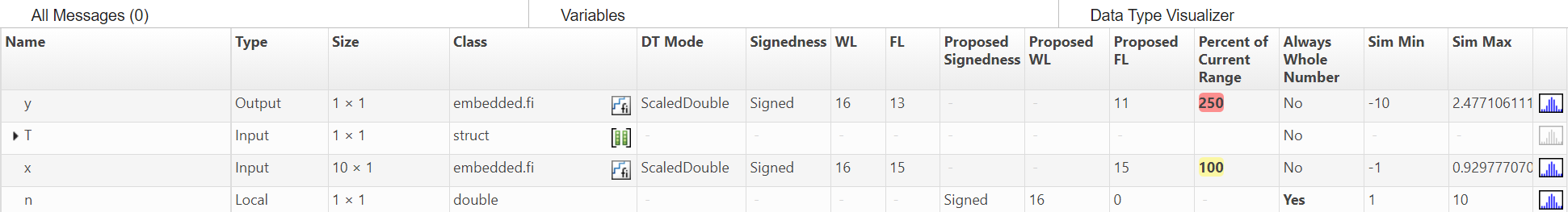6. 将测试脚本更新为对 `y` 使用具有建议的新类型的定标双精度。在 `myTypes.m` 中，对于 `'scaled'` case，使用 ` T.y = fi([],true,16,11,'DataType','ScaledDouble')`

7. 重新运行测试脚本。

现在没有出现溢出。

#### 为建议的定点类型生成代码

1. `myTypes.m` 中，对于 `'fixed'` case，使用 `T.y = fi([],true,16,11)`

2. 更新测试脚本 `mysum_test`，以使用 `T = mytypes('fixed');`

3. 运行测试脚本，然后点击“查看报告”链接以查看生成的 C 代码。

```short mysum(const short x) { short y; int n; int i; int i1; int i2; int i3; y = 0; for (n = 0; n < 10; n++) { i = y << 4; i1 = x[n]; if ((i & 1048576) != 0) { i2 = i | -1048576; } else { i2 = i & 1048575; } if ((i1 & 1048576) != 0) { i3 = i1 | -1048576; } else { i3 = i1 & 1048575; } i = i2 + i3; if ((i & 1048576) != 0) { i |= -1048576; } else { i &= 1048575; } i = (i + 8) >> 4; if (i > 32767) { i = 32767; } else { if (i < -32768) { i = -32768; } } y = (short)i; } return y; }```

默认情况下，`fi` 算术在溢出和最接近舍入时使用饱和，导致代码效率低下。

#### 修改 fimath 设置

1. `myTypes.m` 中，添加 `'fixed2'` case：

``` case 'fixed2' F = fimath('RoundingMethod', 'Floor', ... 'OverflowAction', 'Wrap', ... 'ProductMode', 'FullPrecision', ... 'SumMode', 'KeepLSB', ... 'SumWordLength', 32, ... 'CastBeforeSum', true); T.x = fi([],true,16,15,F); T.y = fi([],true,16,11,F); ```

提示

您可以使用 MATLAB 编辑器中的插入 fimath 选项，而不是手动输入 `fimath` 属性。有关详细信息，请参阅Building fimath Object Constructors in a GUI

2. 更新测试脚本以使用 `'fixed2'`、运行脚本，然后查看生成的 C 代码。

```short mysum(const short x) { short y; int n; y = 0; for (n = 0; n < 10; n++) { y = (short)(((y << 4) + x[n]) >> 4); } return y; }```

生成的代码更加高效，但是 `y` 被移位以与 `x` 对齐，失去了 4 位精度。

3. 为了解决这种精度损失问题，将 `y` 的字长更新为 32 位，并保持 15 位的精度以与 `x` 对齐。

`myTypes.m` 中，添加 `'fixed32'` case：

``` case 'fixed32' F = fimath('RoundingMethod', 'Floor', ... 'OverflowAction', 'Wrap', ... 'ProductMode', 'FullPrecision', ... 'SumMode', 'KeepLSB', ... 'SumWordLength', 32, ... 'CastBeforeSum', true); T.x = fi([],true,16,15,F); T.y = fi([],true,32,15,F); ```

4. 更新测试脚本以使用 `'fixed32'` 并运行脚本以再次生成代码。

现在，生成的代码非常高效。

```int mysum(const short x) { int y; int n; y = 0; for (n = 0; n < 10; n++) { y += x[n]; } return y; }```